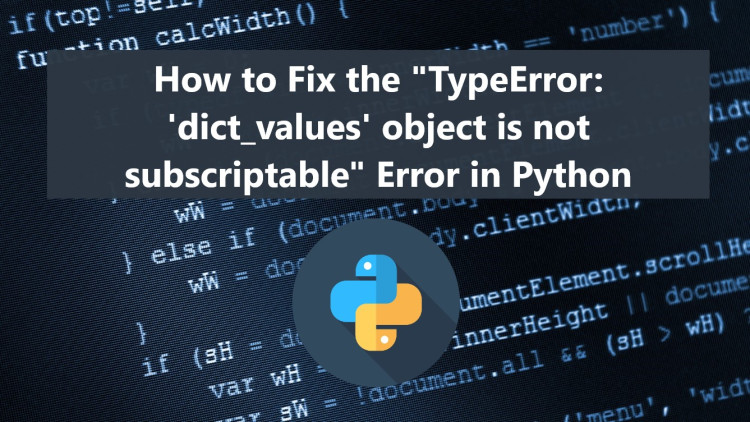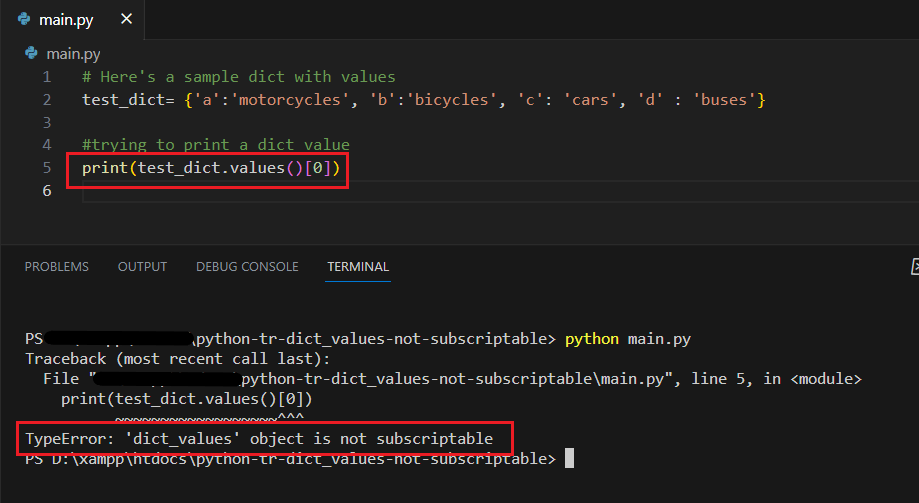# How to Fix the "TypeError: 'dict_values' object is not subscriptable" Error in PythonIf you are facing a Python Type Error that raises TypeError: 'dict_values' object is not subscriptable, this article will help you to understand why the error occurs and how to solve it.

## What is Python dict_values?

The dict_values is a built-in method of a Dictionary in Python. It can be initiated or called by using the values() method. The method will return dict_values of certain Dictionary values. The values() do not take any parameters and do not return the dictionary's keys.

For Example, we have the following Dictionary:

`sample = {'a': 'foo', 'b':'bar'}`

If you try to print the dictionary values using the values() method:

`sample = {'a': 'foo', 'b':'bar'}print(sample.values())`

This will execute and output the following:

```dict_values(['foo', 'bar'])
```

## Why does the "TypeError: 'dict_values' object is not subscriptable" Error occur?

The "TypeError: 'dict_values' object is not subscriptable" occurs when we are trying to output a value from the dict_values using an index. The following script is an example Python script that simulates the "type error".

`# Here's a sample dict with valuestest_dict= {'a':'motorcycles', 'b':'bicycles', 'c': 'cars', 'd' : 'buses'} #trying to print a dict valueprint(test_dict.values()) `

The script will result in the following:The error occurred because the values() method returns dict_values and we are trying to output a value from the dictionary using a list index which is not possible.

## Python's "TypeError: 'dict_values' object is not subscriptable" [Solved]

Fortunately, this Python Type Error can be easily solved using the built-in method called list(). Using this method, we can list the dictionary values where we can get the values using an index. To do that, we can simply get the dictionary values [dict_values] using the values() method and convert them to a list using the list() method. Check out the sample fixed script that I provided below.

### Solution #1:

`# Here's a sample dict with valuestest_dict= {'a':'motorcycles', 'b':'bicycles', 'c': 'cars', 'd' : 'buses'} #trying to print a dict valueprint(list(test_dict.values())) `

Result

### Solution #2:

`# Here's a sample dict with valuestest_dict= {'a':'motorcycles', 'b':'bicycles', 'c': 'cars', 'd' : 'buses'} #trying to print a dict valuetd_vals = test_dict.values()td_list = list(td_vals)print(td_list) `

Result

### Solution #3:

`# Here's a sample dict with valuestest_dict= {'a':'motorcycles', 'b':'bicycles', 'c': 'cars', 'd' : 'buses'} #trying to print a dict valuetd_vals = test_dict.values()td_list = list(td_vals)print(td_list[:-1]) `

Result

There you go! That is how we can fix or solve the "TypeError: 'dict_values' object is not subscriptable" error of Python. I hope this article helps you to understand why the said Python Type Error occurs and how to solve it.

That's it! That is how to Solve the Python "TypeError: 'dict_values' object is not subscriptable" error article will help you with what you are looking for and will be useful to prevent this kind of error for your future Python Projects.

Explore more on this website for more Tutorials and Free Source Codes.